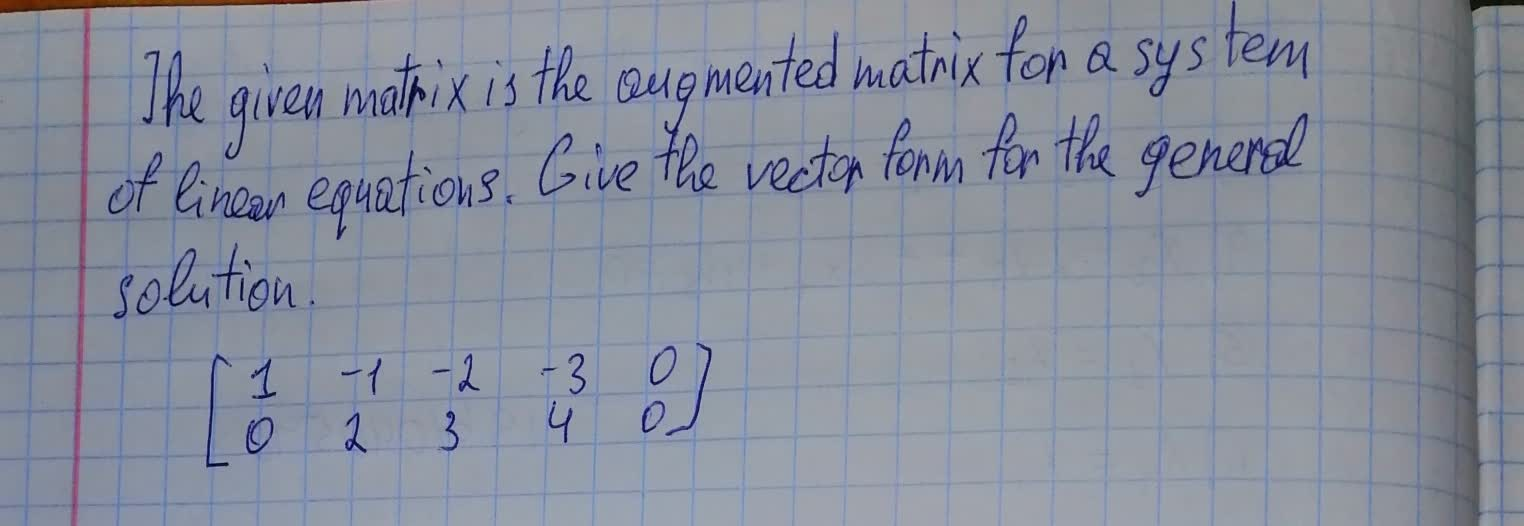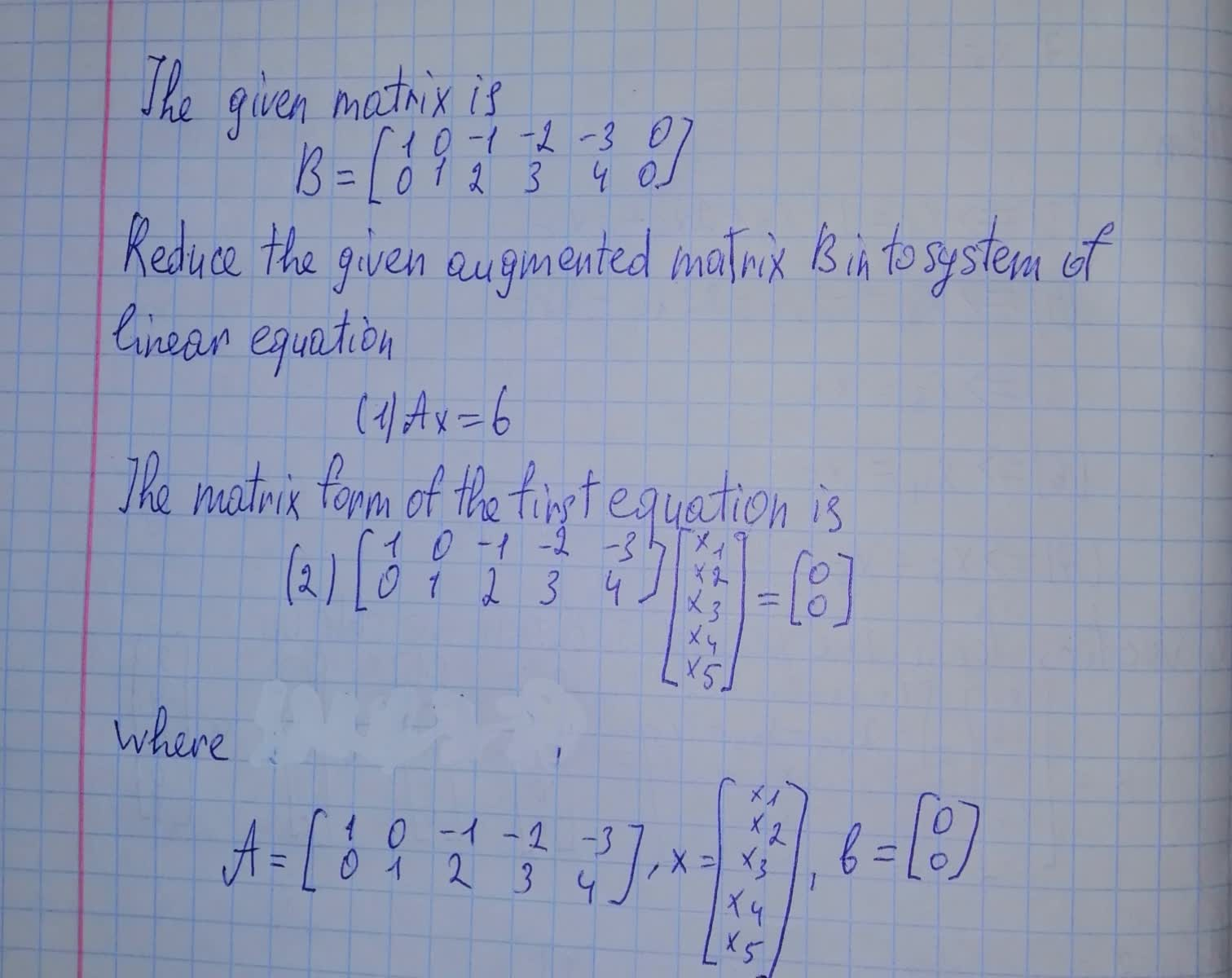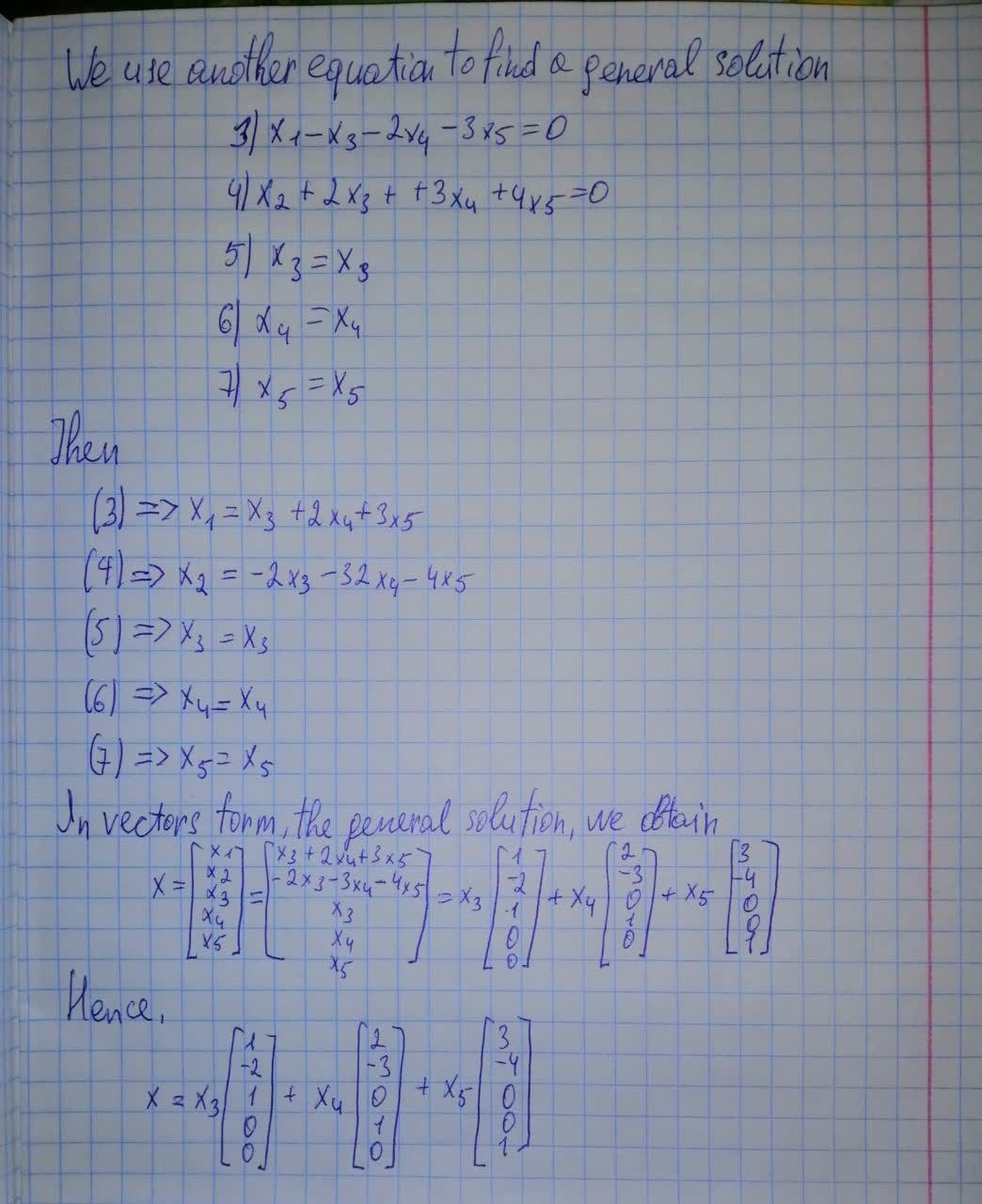Question# The given matrix is the augmented matrix for a system of linear equations. \begin{bmatrix}1&0&-1&-2&-3&0 \\0&1&2&3&4&0 \end{bmatrix}

Forms of linear equations
ANSWEREDThe given matrix is the augmented matrix for a system of linear equations. Give the vector form for the general solution. $$\begin{bmatrix}{1}&{0}&-{1}&-{2}&-{3}&{0}\\{0}&{1}&{2}&{3}&{4}&{0}\end{bmatrix}$$